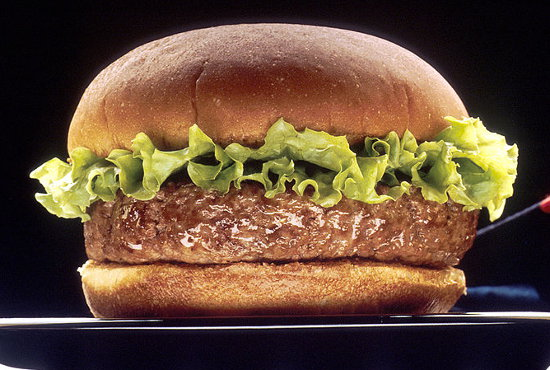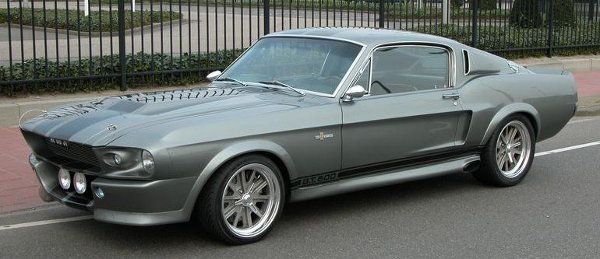# Compound Interest

Compound Interest is when the interest is added to the principal at regular intervals.
The interest is said to be compounded.

The Compound Interest rule is:

A = P ( 1 + i ) n

where
A = amount after investment time period
P = principal or amount invested
i = interest rate (when the interest is compounded or added to the bank account; as a decimal)
n = number of times that the interest is compounded or added to the account)

This rule is really a series of Simple Interest calculations (Interest = Principal × Interest Rate × Time) as shown in the following long example. A shorter method using the Compound Interest rule is also shown below.

## Example One - Compound Interest

I have \$100 in a savings bank account. The bank pays 10% interest each year. This interest is added to my bank account each year. How much will I have after 3 years?

Answer (LONG METHOD WITHOUT THE RULE):
These calculations are a series of yearly simple interest calculations.
Interest after 1st year
= Principal × Interest Rate × Time
= \$100 × 10% × 1yr
= 100 × 0.1 × 1
= \$10

Amount after 1st year
= \$100 + \$10
= \$110

Interest after 2nd year
= \$110 × 10% × 1yr
= \$11

Amount after 2nd year
= \$110 + \$11
= \$121

Interest after 3rd year
= \$121 × 10% × 1yr
= \$12.10

Amount after 3rd year
= \$121 + \$12.10
= \$133.10

Answer (SHORT METHOD WITH THE RULE):
P = \$100
i = 10% = 0.1
n = 3 yr

A = P × ( 1 + i ) n
A = 100 × ( 1 + 0.1 ) 3
A = \$133.10

## Example Two - Compounded Yearly

I have \$100 in my bank account. The bank pays interest of 12% p.a. (per annum = each year) compounded yearly. How much will I have after 5 years?

P = \$100
r = 12% = 0.08
n = 5 yr

A = P × ( 1 + i ) n
A = 100 × ( 1 + 0.12 ) 5
A = \$176.23

## Question - Compounded Yearly

I have \$6000 in my bank account. The bank pays 5 14 % interest p.a. If my investment is compounded annually, how much will I have after 3 years?

\$6995.48

## Example Three - Compounded Monthly

I have \$100 in my bank account. The bank pays interest of 12% p.a. compounded monthly. How much will I have after 5 years?

P = \$100
i = 12% ÷ 12 months = 1% = 0.01
n = 5 yr × 12 months = 60

A = P × ( 1 + i ) n
A = 100 × ( 1 + 0.01 ) 60
A = \$181.67

Notice that you calculate compound interest monthly, you divide i by 12 and multiply n by 12.

## Question - Compounded Monthly

I have \$600 in my bank account. The bank pays 9% interest p.a. If my investment is compounded monthly, how much will I have after 3 years?

\$785.19

## Example Four - Compounded Quarterly

Compounded Quarterly is compounding 4 times per year.

I have \$100 in my bank account. The bank pays interest of 12% p.a. compounded quarterly. How much will I have after 5 years?

P = \$100
i = 12% ÷ 4 = 3% = 0.03
n = 5 yr × 4 quarters = 20

A = P × ( 1 + i ) n
A = 100 × ( 1 + 0.03 ) 20
A = \$180.61

Notice that you calculate compound interest quarterly, you divide i by 4 and multiply n by 4.

## Question - Compounded Quarterly

I have \$680 in my bank account. The bank pays 8.4% interest p.a. If my investment is compounded quarterly, how much will I have after 2 years?

\$803

## Example Five - Compounded Weekly

I have \$100 in my bank account. The bank pays interest of 12% p.a. compounded weekly. How much will I have after 5 years?

P = \$100
i = 12% ÷ 52 = 0.0023
n = 5 yr × 52 weeks = 260

A = P × ( 1 + i ) n
A = 100 × ( 1 + 0.0023 ) 260
A = \$182.09

Notice that you calculate compound interest weekly, you divide i by 52 and multiply n by 52.

## Question - Compounded Weekly

I have \$600 in my bank account. The bank pays 5.2% interest p.a. If my investment is compounded weekly, how much will I have after 3 years?

\$701.24

## Maths Fun - Which is the Better Investment?

Look at Examples 2, 3, 4 and 5. The principal, the interest rate and the time of investment are the same. Only the number of times that the interest is compounded (i.e. calculated and added to the bank account) differs. How would interest compounded daily compare with these?

## Example Six - InflationInflation is the change of money's value over time. A Big Mac costs \$4 now. If our country's inflation rate is a steady 3% each year, what will the same Big Mac cost 10 years into the future?

P = \$40
i = 3% = 0.03
n = 10 yr

A = P × ( 1 + i ) n
A = 4 × ( 1 + 0.03 ) 10
A = \$5.38

## Did You Know That...?

The cheapest Big Mac is in Ukraine; the most expensive is in Switzerland.

## Example Seven - AppreciationAppreciation is the increase in an item's value over time. A 1967 Shelby Mustang cost \$4000 when new. Its annual appreciation rate is approximately 9%. What would you expect to pay for it 2017?

P = \$4000
i = 9% = 0.09
n = 50 yr

A = P × ( 1 + i ) n
A = 4000 × ( 1 + 0.09 ) 50
A = \$297 430

## Example Eight - Population Growth

The Philippines has a fast-growing population with an annual increase of approximately 1.7 %. If its population in 2012 was 98 million, what is the expected population in 2020?

P = 98 000 000
i = 1.7% = 0.017
n = 8 yr

A = P × ( 1 + i ) n
A = 98 000 000 × ( 1 + 0.017 ) 8
A = 112 148 559 people approximately

## Question

In Example 7, the Shelby Mustang increases in price by approximately 9% p.a., but inflation is about 3% of this. Its real increase is 6% p.a. compounded yearly. Rob the rev-head has \$150 000 that he wants to invest in either a Shelby Mustang or leave it in the bank at 5.9% compounded weekly. Which is the better investment?